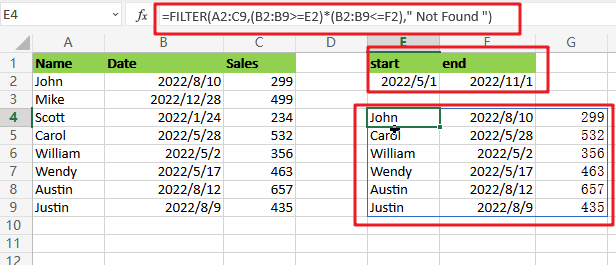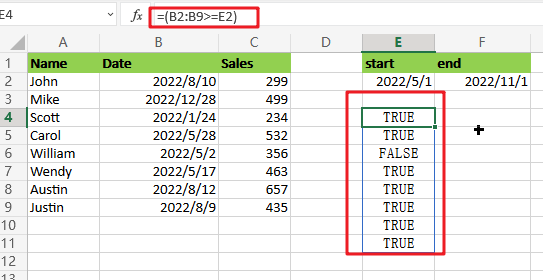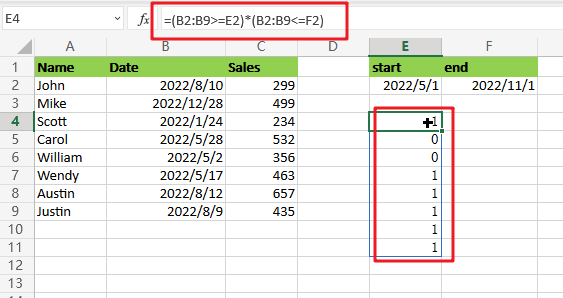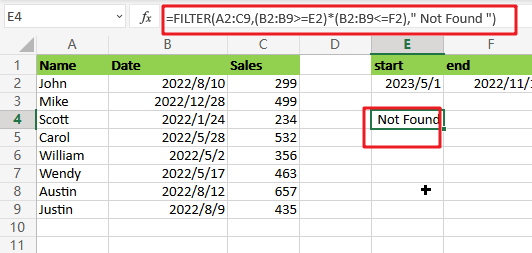# Filter Data between Date Values

This post will guide you how to filter data between two given date values using FILTER function in Microsoft Excel.The General Formula is as follow:

`=FILTER(total_data,(date_range>=start_date)*( date_range <= end_date),"Not Found")`

## Summary:

You can filter data to include only records between two dates with the FILTER function. For example, E4 uses this logic:

`=FILTER(A2:C9,(B2:B9>=E2)*(B2:B9<=F2)," Not Found ")`The range of dates that the filter returns is `2022/5/1- 2022/11/1`, inclusive.

## Clarification:

We can extract data based on a logical test created with our boolean logic expression with this filter function. The array value is provided as A2:C9, containing all the full sets without headers. It also includes two comparisons to see if any part matches what’s expected in order for something else to be returned.

The included Filter Function relies heavily upon one’s ability to create expressions, and the strongest aspect is to evaluate them!

`=(B2:B9>=E2)*(B2:B9<=F2)`

The left expression checks if dates are greater than or equal to their “`start`” date in E2. This is an example of Boolean logic, and it has been joined with another function, which creates an AND relationship between both operations, which means they can’t be true at the same time! The right-hand side also involves some Date math, so this formula does not just return any old value but rather something meaningful like ‘After’.

We have result after applying logical expressions:For this experiment, there are eleven values in each set of parentheses. The multiplication operation coerced the TRUE FALSE values into 1s, and 0’s so that the final result is an array-like these:The six 1s in this array represent the dates we will have data to analyze. Our FILTER function uses these values, which filter out any rows without an equal or higher value at either end, making them easier for us!

If a matching piece of data is not found, this value will be set to ” `Not Found `“.### Related Functions

• Excel Filter function
The Excel  FILTER function extracts matched records from a collection of data using one or more logical checks. The include argument specifies logical tests, which might encompass a wide variety of formula conditions.==FILTER(array,include,[if empty])…